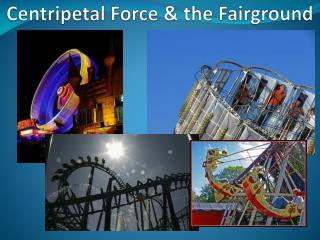# Centripetal Force & the Fairground - PowerPoint PPT PresentationDownload PresentationCentripetal Force & the Fairground

Download Presentation## Centripetal Force & the Fairground

- - - - - - - - - - - - - - - - - - - - - - - - - - - E N D - - - - - - - - - - - - - - - - - - - - - - - - - - -
##### Presentation Transcript

1. Centripetal Force & the Fairground

2. Learning Objectives Book Reference : Pages 28-29 Centripetal Force & the Fairground • To apply what we have learnt about circular motion to rides at the fairground • To consider three particular cases of motion: • The big dipper • The long swing • The “centrifuge” wall of death

3. At the bottom of a big dipper you are pushed into your seat and feel “heavier”... Centripetal Force: Big Dippers 1 Centre of curvature S velocity mg

4. At the bottom of the dip at speed v with radius r, Resolving the vertical forces: • S – mg = mv2 / r • S = mg + mv2 / r • The “extra” weight you experience when feeling “heavy” is given by the centripetal force Centripetal Force: Big Dippers 2

5. Consider a person of mass m on a very long swing of length r Centripetal Force: The Long Swing 1 To winch r Fixed point Initial position S Velocity mg

6. As the swing is released we can consider the conservation of energy, loss in potential energy is the gain in the kinetic energy • mgh = ½mv2 v2 = 2gh • The passenger is on a circular path with radius r. At the bottom of the swing the support force S acts against the person’s weight mg. This provides the centripetal force: • S – mg = mv2/r Centripetal Force: The Long Swing 2

7. Substituting for v2: • S – mg = mv2/r • S – mg = 2mgh/r • S = mg + 2mgh/r • The person “feels heavier” by 2mgh/r, if the swing drops through 90°, then the extra support force is twice the persons weight (mg) Centripetal Force: The Long Swing 3

8. Consider a fairground ride which spins fast enough to keep you in place even when upside down at the top of the ride.... Centripetal Force: The wall of death 1 Velocity Reaction R & weight mg Rotation

9. The wheel turns fast enough to keep the passenger in position as they pass over the top. • At the top, the reaction R acts downwards and together with the weight provides the centripetal force: • mg + R = mv2/ r • R = mv2/ r – mg • At a certain speed v0such that v02 = gr, then the reaction from the wall will be zero Centripetal Force: The wall of death 2

10. A car on a big dipper starts from rest and descends though 45m into a dip which has a radius of curvature of 78m. Assuming that air resistance & friction are negligible. Calculate: • The speed of the car at the bottom of the dip • The centripetal acceleration at the bottom of the dip • The extra force on a person with a weight of 600N in the train Problems 1

11. A swing at a fair has a length of 32m. A passenger of mass 69kg falls from the position where the swing is horizontal. Calculate: • The speed of the person at the lowest point • The centripetal acceleration at the lowest point • The support force on the person at the lowest point Problems 2

12. A wall of death ride at the fairground has a radius of 12m and rotates once every 6s. Calculate: • The speed of rotation at the perimeter of the wheel • The centripetal acceleration of a person on the perimeter • The support force on a person of mass 72kg at the highest point Problems 3